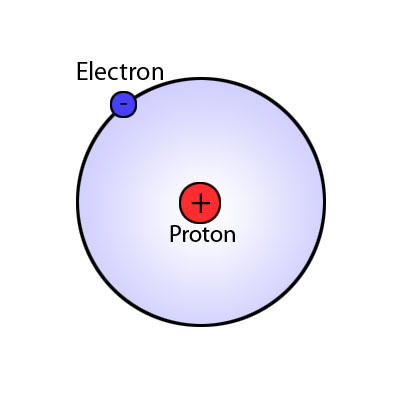# How many valence electrons does hydrogen have?

Apr 15, 2014

Hydrogen has 1 valence electron.

#### Explanation:

Hydrogen is in the first row of the Periodic Table. Elements in the first row are filling their $\text{1s}$ orbitals.

Since hydrogen is the first element, its electron configuration is $1 {\text{s}}^{1}$. It has only one electron in its valence shell.

Valence electrons are the outermost electrons or electrons in the largest energy level.

Hydrogen has only one electron, so that electron is a valence electron.Example

How many electrons does helium have?

Solution

Helium is also in the first row of the Periodic Table. It is filling its 1s orbital.

Since helium is the second element, it has two electrons. Its electron configuration is ${\text{1s}}^{2}$.Helium has two electrons in its valence shell.

Here is a video that gives further explanation of this topic.

Video from: Noel Pauller